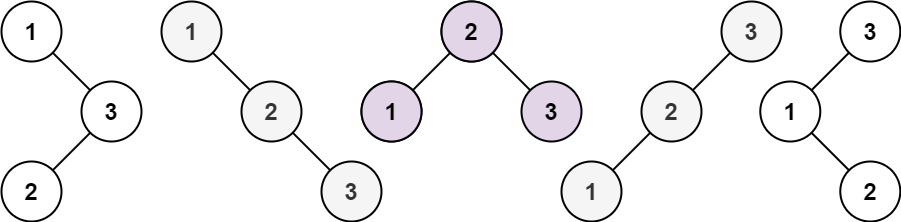# 96. Unique Binary Search Trees

## 96. Unique Binary Search Trees

Given an integer `n`, return the number of structurally unique BST's (binary search trees) which has exactly `n` nodes of unique values from `1` to `n`.

Example 1:```Input: n = 3
Output: 5
```

Example 2:

```Input: n = 1
Output: 1
```

Constraints:

• `1 <= n <= 19`

### The solution in C++ using DP

``````           3                          2                         1
/ \                        / \                      /   \
numTrees(2) numTrees(0)    numTrees(1) numTrees(1)   numTrees(0) numTrees(2)
i = 3                      i = 2                     i = 1

i
=>              /   \
numTrees(i-1)	numTrees(n-i)``````
class Solution {
public:
int dp;
int numTrees(int n) {
dp=1;
dp=1;
if(n <= 1){
return dp[n];
}

for(int i = 1i <= ni++) {
if(dp[i-1]==0dp[i-1]=numTrees(i-1);
if(dp[n-1]==0dp[n-i]=numTrees(n-i);
dp[n] += dp[i-1]* dp[n-i];
}

return dp[n];
}
};